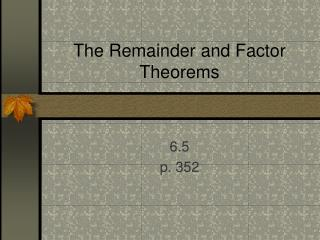DownloadDownload PresentationThe Remainder and Factor Theorems

# The Remainder and Factor Theorems

Download Presentation## The Remainder and Factor Theorems

- - - - - - - - - - - - - - - - - - - - - - - - - - - E N D - - - - - - - - - - - - - - - - - - - - - - - - - - -
##### Presentation Transcript

1. The Remainder and Factor Theorems 6.5 p. 352

2. When you divide a Polynomial f(x) by a divisor d(x), you get a quotient polynomial q(x) with a remainder r(x) written:f(x) = q(x) + r(x)d(x) d(x)

3. Polynomial Long Division: • You write the division problem in the same format you would use for numbers. If a term is missing in standard form …fill it in with a 0 coefficient. • Example: • 2x4 + 3x3 + 5x – 1 = • x2 – 2x + 2

4. 2x2 2x4 = 2x2 x2

5. 2x2 +7x +10 -( ) 2x4 -4x3 +4x2 7x3 - 4x2 +5x -( ) 7x3 - 14x2 +14x 10x2 - 9x -1 7x3 = 7x x2 -( ) 10x2 - 20x +20 11x - 21 remainder

6. The answer is written: • 2x2 + 7x + 10 + 11x – 21 x2 – 2x + 2 • Quotient + Remainder over divisor

7. Now you try one! • y4 + 2y2 – y + 5 = y2 – y + 1 • Answer: y2 + y + 2 + 3 y2 – y + 1

8. Remainder Theorem: • If a polynomial f(x) is divisible by (x – k), then the remainder is r = f(k). • Now you will use synthetic division (like synthetic substitution) • f(x)= 3x3 – 2x2 + 2x – 5 • Divide by x - 2

9. f(x)= 3x3 – 2x2 + 2x – 5 Divide by x - 2 • Long division results in ?...... • 3x2 + 4x + 10 + 15 x – 2 • Synthetic Division: • f(2) = 3 -2 2 -5 2 6 8 20 3 4 10 15 Which gives you: + 15 x-2 3x2 + 10 + 4x

10. Synthetic Division Practice 1 • Divide x3 + 2x2 – 6x -9 by (a) x-2 (b) x+3 • (a) x-2 • 1 2 -6 -9 2 8 4 2 1 4 2 -5 Which is x2 + 4x + 2 + -5 x-2

11. Synthetic Division Practice cont. • (b) x+3 • 1 2 -6 -9 -3 3 9 -3 1 -1 -3 0 x2 – x - 3

12. Factor Theorem: • A polynomial f(x) has factor x-k iff f(k)=0 • note that k is a ZERO of the function because f(k)=0

13. Factoring a polynomial • Factor f(x) = 2x3 + 11x2 + 18x + 9 • Given f(-3)=0 • Since f(-3)=0 • x-(-3) or x+3 is a factor • So use synthetic division to find the others!!

14. Factoring a polynomial cont. • 2 11 18 9 • -3 -15 -9 -6 2 5 3 0 So…. 2x3 + 11x2 + 18x + 9 factors to: (x + 3)(2x2 + 5x + 3) Now keep factoring (bustin ‘da ‘b’) gives you: (x+3)(2x+3)(x+1)

15. Your turn! • Factor f(x)= 3x3 + 13x2 + 2x -8 • given f(-4)=0 • (x + 1)(3x – 2)(x + 4)

16. Finding the zeros of a polynomial function • f(x) = x3 – 2x2 – 9x +18. • One zero of f(x) is x=2 • Find the others! • Use synthetic div. to reduce the degree of the polynomial function and factor completely. • (x-2)(x2-9) = (x-2)(x+3)(x-3) • Therefore, the zeros are x=2,3,-3!!!

17. Your turn! • f(x) = x3 + 6x2 + 3x -10 • X=-5 is one zero, find the others! • The zeros are x=2,-1,-5 • Because the factors are (x-2)(x+1)(x+5)

18. Assignment